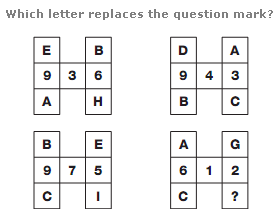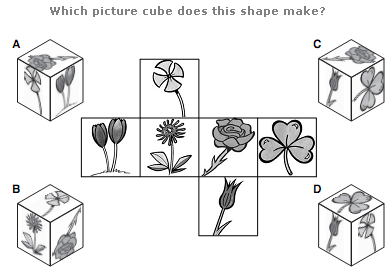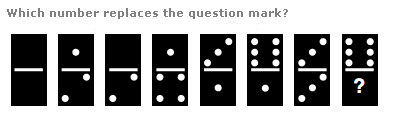# Puzzles - Logical puzzles

### Exercise :: Logical puzzlesAnswer : F Explanation : In each diagram, convert each letter to its numerical value, and read the top and bottom pairs of letters as complete 2 digit values. Multiply these values together to give the 3 digit result written in the centre spaces.Answer : C Explanation : [NIL]Answer : 3 Explanation : As you move from left to right, the spot total on each domino increases by 3 then decreases by 1 alternately.